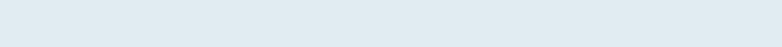# Count Unique Text Values in a Column with Criteria

## Query

We have two columns Product Manager (PM) and Vendor Name (VN). Our task is to find the unique count of VN who bought from a PM irrespective of number times they purchased. The purpose is to find how many vendors a PM handle.

## Formula to Implement:

{=SUM(N(FREQUENCY(IF(B4:B14=F4,MATCH(C4:C14,C4:C14,0)),ROW(C4:C14)-ROW(B3))>0))}

• While writing this I used Office 365, however, anyone using Excel 2016, Excel 2013 and even prior editions of Excel should be able to follow along with the content.

## Functions applicable:

You will be creating an Array formula along with the following Excel functions:

• SUM – Adding multiple numeric arguments together
• N – Code number by converting a specific reference
• FREQUENCY – Total number of occurrences of a value in a given bin of data set.
• IF – Returns TRUE or FALSE based on a valid test of criteria.
• MATCH – Finds row or column of a given value containing in a data set (Excluding duplicates)
• ROW – Number of rows of a cell range or array formula.

## Formula Explanation (Step by Step)

Excel start calculating from the innermost parenthesis and then work outward. You can also evaluate or debug a formula using the F9 shortcut key.

Let’s break our formula into multiple small steps to understand it’s application.

First FormulaIF(B4:B14=F4,MATCH(C4:C14,C4:C14,0)

Syntax

IF(logical_test, [value_if_true], [value_if_false])
MATCH(lookup_value, lookup_array, [match_type])

Step 1 IF(B4:B14=F4)                    ‘compare two text

Underlined Expression

{“Tilley”;”Moss“;”Popiel”;”Popiel”;”Moss“;”Furn”;”Tilley”;”Moss“;”Furn”;”Moss”;”Furn”}= “Moss”

The Excel check a cell range (B4:B14) within an array constant {} and compare with “Moss” (F4 – cell to evaluate); and returns TRUE if it matches and FALSE if it does not.

Result

{FALSE;TRUE;FALSE;FALSE;TRUE;FALSE;FALSE;TRUE;FALSE;TRUE;FALSE}

Moss occurs three times (TRUE) in the given cell range as per the above result.

Step 2 MATCH(C4:C14,C4:C14,0)        ‘Find row number of each unique text

Result

{1;2;3;4;1;4;2;4;4;2;4}

Match function returns the ROW number within the selected cell range (C4:C14) but assigns same row number in case the text is not unique.
For example, there are five occurrences of Stout represented by row number ‘4’; Stout first occurrence in the list is 4th (cell C7).

Step 3 – IF(B4:B14=F4,MATCH(C4:C14,C4:C14,0)               ‘Return row number if TRUE

Now IF function evaluates both results as mention in Step 1 and Step 2. Moreover, returns row number [value_if_true] only if the logical test in Step 1 is TRUE (check IF syntax) otherwise return the same value as you have not chosen the 2nd optional parameter of the function [value_if_false].

Result

{FALSE;2;FALSE;FALSE;1;FALSE;FALSE;4;FALSE;2;FALSE}

So now the Excel only returns the row number of those Vendors (Column C) only that are associated with “Moss” (Column B).

Second Formula – ROW(C4:C14)-ROW(B3)      ‘Create a numeric sequence for bins

Step 4ROW(C4:C14)

Result

{4;5;6;7;8;9;10;11;12;13;14}

Returns row number of each cell in range ‘C4:C14’.

Step 5 – ROW(B3)

Result – {3}

Returns row number of cell B3.

Formula result

The formula subtract two array constants and returns a new one.

{4;5;6;7;8;9;10;11;12;13;14} – {3} = {1;2;3;4;5;6;7;8;9;10;11}

The new Row number is used as bins in the Excel FREQUENCY function of your next formula.

Integrate First Two Formula

FREQUENCY(IF(B4:B14=F4,MATCH(C4:C14,C4:C14,0)),ROW(C4:C14)-ROW(B3))      ‘Occurrence for each bins

Syntax -FREQUENCY(data_array, bins_array)

After replacing results based on first two formula (Step 1 to 5).

FREQUENCY({FALSE;2;FALSE;FALSE;1;FALSE;FALSE;4;FALSE;2;FALSE},{1;2;3;4;5;6;7;8;9;10;11})Step 6

Result

{1;2;0;1;0;0;0;0;0;0;0;0}

FREQUENCY function check and returns number of occurrence like 2 occur twice in Step 3 that represent ‘Reimers’.

Reimers occurs twice whereas Loman and Stout occur only once with Moss.

Third Formula

Step 7

=SUM(N({1;2;0;1;0;0;0;0;0;0;0;0}>0))

First, check each array elements if more than ‘Zero‘. Returns TRUE if it is more and FALSE if it does not.

N{TRUE;TRUE;FALSE;TRUE;FALSE;FALSE;FALSE;FALSE;FALSE;FALSE;FALSE;FALSE}

Then convert TRUE and FALSE to 1 and 0 respectively.

Step 8

=SUM({1;1;0;1;0;0;0;0;0;0;0;0})

Now you add all elements of array and returns 3.

## Conclusion

The Excel formula returns a count of unique texts (no duplicate) based on the given condition of the first column.